https://doi.org/10.5194/amt-13-4277-2020
https://doi.org/10.5194/amt-13-4277-2020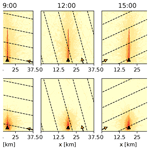# Three-dimensional radiative transfer effects on airborne and ground-based trace gas remote sensing

Marc Schwaerzel, Claudia Emde, Dominik Brunner, Randulph Morales, Thomas Wagner, Alexis Berne, Brigitte Buchmann, and Gerrit Kuhlmann
Abstract

Air mass factors (AMFs) are used in passive trace gas remote sensing for converting slant column densities (SCDs) to vertical column densities (VCDs). AMFs are traditionally computed with 1D radiative transfer models assuming horizontally homogeneous conditions. However, when observations are made with high spatial resolution in a heterogeneous atmosphere or above a heterogeneous surface, 3D effects may not be negligible. To study the importance of 3D effects on AMFs for different types of trace gas remote sensing, we implemented 1D-layer and 3D-box AMFs into the Monte carlo code for the phYSically correct Tracing of photons In Cloudy atmospheres (MYSTIC), a solver of the libRadtran radiative transfer model (RTM). The 3D-box AMF implementation is fully consistent with 1D-layer AMFs under horizontally homogeneous conditions and agrees very well (<5 % relative error) with 1D-layer AMFs computed by other RTMs for a wide range of scenarios. The 3D-box AMFs make it possible to visualize the 3D spatial distribution of the sensitivity of a trace gas observation, which we demonstrate with two examples. First, we computed 3D-box AMFs for ground-based multi-axis spectrometer (MAX-DOAS) observations for different viewing geometry and aerosol scenarios. The results illustrate how the sensitivity reduces with distance from the instrument and that a non-negligible part of the signal originates from outside the line of sight. Such information is invaluable for interpreting MAX-DOAS observations in heterogeneous environments such as urban areas. Second, 3D-box AMFs were used to generate synthetic nitrogen dioxide (NO2) SCDs for an airborne imaging spectrometer observing the NO2 plume emitted from a tall stack. The plume was imaged under different solar zenith angles and solar azimuth angles. To demonstrate the limitations of classical 1D-layer AMFs, VCDs were then computed assuming horizontal homogeneity. As a result, the imaged NO2 plume was shifted in space, which led to a strong underestimation of the total VCDs in the plume maximum and an underestimation of the integrated line densities that can be used for estimating emissions from NO2 images. The two examples demonstrate the importance of 3D effects for several types of ground-based and airborne remote sensing when the atmosphere cannot be assumed to be horizontally homogeneous, which is typically the case in the vicinity of emission sources or in cities.

Share
Dates
1 Introduction

Ground-based, space-based and airborne remote sensing of air pollutants and greenhouse gases from scattered sunlight are increasingly used for air pollutant monitoring and for source detection and emission estimation . The most commonly applied trace gas retrieval method in the ultraviolet, visible and near-infrared spectral range is differential optical absorption spectroscopy (DOAS) , which fits absorption cross sections of a trace gas to the measured spectra. The result of the DOAS analysis is a slant column density (SCD), which is the integrated trace gas concentration along the optical path of the sunlight scattered towards the spectrometer. The optical path depends on the illumination and viewing geometry, on absorption and scattering by air molecules, aerosols and clouds, and surface reflectance.

A physically more meaningful quantity that is independent of the measurement geometry is the vertical column density (VCD), which is the integrated trace gas concentration from the ground to the top of the atmosphere. The ratio between SCD and VCD is called air mass factor (AMF) , which can be computed with a radiative transfer model (RTM). To account for the vertical variability in atmospheric properties, AMFs are computed for discrete vertical layers (layer AMFs) assuming horizontal homogeneity . In the past decades, numerous RTMs have been developed with the possibility to calculate one-dimensional layer AMFs . The computation of layer AMFs is implemented in most trace gas retrieval algorithms for satellite and ground-based observations applied today . An alternative method is direct fitting, which is used in few algorithms (e.g., Lerot et al.2010).

Layer AMFs assume horizontal homogeneity, which is not valid when the parameters affecting scattering and absorption along the path of the photons vary also horizontally, for example, in limb geometry near the polar vortex or in the presence of clouds . Horizontal homogeneity is usually a valid assumption in coarse-resolution trace gas remote sensing from satellites, where small-scale horizontal variability is averaged over a large pixel size. It is, however, often not valid for ground-based or airborne trace gas remote sensing at high resolution in polluted environments such as cities . This is particularly true for nitrogen dioxide (NO2), which has high spatial and temporal variability due to its short lifetime . Other parameters affecting the path of the measured photons like surface reflectance and aerosol distributions may also have high spatial variability in cities.

To account for horizontal inhomogeneity, one-dimensional (1D) layer AMFs need to be extended to three-dimensional (3D) box AMFs. Notice that in previous studies (e.g., Rozanov and Rozanov2010) 1D-layer AMFs were sometimes referred to as box AMFs. In this study, we will use the terms 1D-layer and 3D-box AMFs to clearly distinguish between them. The 3D-box AMFs can be implemented most easily in radiative transfer models that compute the paths of many photons using a Monte Carlo approach to solve the radiative transfer equation . In this study, we implemented both 1D-layer and 3D-box AMFs in the MYSTIC solver of the libRadtran RTM . The implementation was evaluated against the results of a RTM comparison study . Finally, the advantage and necessity of using 3D-box AMFs is demonstrated for a range of realistic ground-based and airborne remote sensing scenarios.

2 Methods

## 2.1 Air mass factors

Atmospheric trace gases can be measured with ground-, aircraft- and space-based spectrometers that measure solar irradiance scattered into the line of sight of the instrument (see Fig. 1). In the case of aircraft- and space-based observations, a large fraction of the measured photons usually travels along a main path (thick dashed line) representing a single reflection at the surface. In the case of ground-based observations, the measured photons must follow a path with at least a single atmospheric scattering into the line of sight of the instrument (except for direct sun observations). Atmospheric scattering and absorption is determined by the distribution and properties of molecules, aerosols and clouds, and it depends on the wavelength of the radiation. Molecular scattering is particularly important in the UV range of the spectrum. Photons are absorbed by the trace gases along the optical path from the sun to the instrument. For a weak absorber such as NO2, the abundance of the trace gas along the mean optical path can be obtained by fitting an absorption cross section to the measured spectrum. Thereby, the mean optical path is the total length of all individual photon paths divided by the number of photons collected by the instrument. The result of the fit is a SCD, which is defined as

$\begin{array}{}\text{(1)}& \mathrm{SCD}=\underset{\mathrm{path}}{\int }c\left(l\right)\mathrm{d}l,\end{array}$

with trace gas concentration c and optical path l. SCDs are not an intrinsic property of the atmosphere, since they depend on the illumination and viewing geometry. Therefore, for most applications, the main quantity of interest is the VCD. It is defined as

$\begin{array}{}\text{(2)}& \mathrm{VCD}=\underset{{z}_{\mathrm{0}}}{\overset{\mathrm{TOA}}{\int }}c\left(z\right)\mathrm{d}z,\end{array}$

with surface elevation z0 and top of the atmosphere, TOA. AMFs, defined as

$\begin{array}{}\text{(3)}& \mathrm{AMF}=\frac{\mathrm{SCD}}{\mathrm{VCD}},\end{array}$

can be computed for a vertically varying atmosphere by dividing the atmosphere in layers with uniform properties (see Fig. 1a and b). The total AMF is then computed from the individual layer AMFs as

$\begin{array}{}\text{(4)}& \mathrm{AMF}=\frac{{\sum }_{k=\mathrm{1}}^{{n}_{z}}{\mathrm{AMF}}_{k}{\mathrm{VCD}}_{k}}{{\sum }_{k=\mathrm{1}}^{{n}_{z}}{\mathrm{VCD}}_{k}},\end{array}$

with AMFk and VCDk being the AMF and VCD in the kth layer, respectively. The total AMF is thus a function not only of the atmospheric properties in each layer but also of the shape of the vertical profile of the trace gas .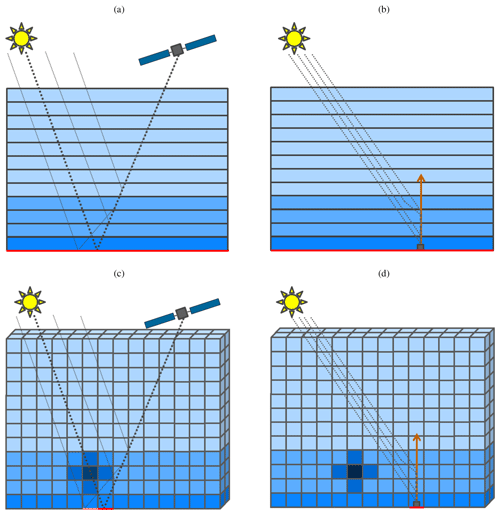Figure 1Illustration of the difference between (a, b) 1D-layer and (c, d) 3D-box AMFs for two scenarios with (a, c) downward-looking spaceborne and (b, d) upward-looking ground-based observations. Selected photon paths are shown as dashed lines. The 1D-layer AMFs implicitly assume horizontally uniform atmospheric and surface properties, whereas 3D-box AMFs fully account for both vertical and horizontal variability.

Similarly, the atmosphere can be divided into boxes in all three dimensions $\left(i,j,k\right)$ with homogeneous optical properties for each box (see Fig. 1c and d). The total AMF can be computed from the 3D-box AMFs $AM{F}_{i,j,k}$ as

$\begin{array}{}\text{(5)}& \mathrm{AMF}=\frac{{\sum }_{i=\mathrm{1}}^{{n}_{x}}{\sum }_{j=\mathrm{1}}^{{n}_{y}}{\sum }_{k=\mathrm{1}}^{{n}_{z}}{\mathrm{AMF}}_{i,j,k}{\mathrm{VCD}}_{i,j,k}}{{\sum }_{k=\mathrm{1}}^{{n}_{z}}{\mathrm{VCD}}_{k}},\end{array}$

where the denominator is a sum over VCDs in k different vertical layers that could, for example, be taken at the location of an instrument or above the ground pixel of an aircraft- or space-based instrument. In this case, the AMF can be interpreted as the instrument sensitivity to the trace gas under investigation for measuring that specific VCD.

## 2.2 Implementation of AMFs in MYSTIC

The libRadtran RTM (available at http://www.libradtran.org, last access: 12 August 2020) can be used to calculate basic radiative quantities with different numerical solvers . One of its solvers is MYSTIC, which uses the Monte Carlo technique to trace individual photons on their way from the source (e.g., sun) to the target (e.g., measurement instrument). Scattering, absorption and reflection processes are treated as random decisions with respective probability distributions. MYSTIC calculates radiative quantities (irradiance, actinic flux at levels, radiance, absorption, emission, actinic flux, photon's path length and air mass factors) in 1D or 3D domains in spherical geometry or in plane-parallel geometry . The 1D-layer and 3D-box AMFs were implemented following the same methodology as in McArtim, which to our knowledge is the only other existing RTM capable of computing 3D-box AMFs . Note that McArtim is no longer actively developed.

AMFs depend on absorption and scattering processes affecting the light path in the atmosphere. AMFs can be readily calculated from the photon paths simulated by a Monte Carlo radiative transfer model. The Monte Carlo technique traces the paths of individual photons by describing the effects of absorption, scattering and reflection as random events with specific probabilities (Mayer2009). To obtain a robust measure of the mean optical path, a large number of photon paths need to be traced.

SCDs, VCDs and AMFs can be computed for the whole atmosphere, for individual vertical layers or for individual 3D boxes. For the general case of an atmospheric box i with constant concentration and optical properties, the AMF can be written as

$\begin{array}{}\text{(6)}& {\mathrm{AMF}}_{i}=\frac{{\mathrm{SCD}}_{i}}{{\mathrm{VCD}}_{i}}=\frac{{\int }_{\mathrm{path}}{c}_{i}\mathrm{d}l}{{\int }_{{z}_{i}}^{{z}_{i+\mathrm{1}}}{c}_{i}\mathrm{d}z}=\frac{{\int }_{\mathrm{path}}\mathrm{d}l}{{h}_{i}}=\frac{{L}_{i}}{{h}_{i}},\end{array}$

where ${L}_{i}={\int }_{\mathrm{path}}\mathrm{d}l$ is the mean optical path within the box of all photons that reach the instrument and hi is the height of the box. Since the 3D-box/1D-layer AMFs are usually simulated for a sensor at a specific location in a three-dimensional model domain, the photons are traced backwards from the sensor towards the sun to increase computational efficiency as described in and . In addition, the commonly used variance reduction method, known as “local estimate”, is applied at each scattering event . The method computes the probability of an individual photon to be scattered into the direction of the sun that is assigned as a weight wn to the photon. The weights of all photons can be summed up to obtain the radiance at the sensor. When a photon is scattered, a weighted photon path length (wnli) is also calculated, where li are the path lengths in each individual box i traversed by the photon before the scattering event. The mean optical path within a box i is then obtained by summing up the weighted photon path lengths of all photons as follows:

$\begin{array}{}\text{(7)}& {L}_{i}=\frac{{\sum }_{n}^{N}{w}_{n}{l}_{i,n}}{{\sum }_{n}^{N}{w}_{n}},\end{array}$

where N is the total number of photons. Li is then divided by the height of the box/layer to obtain the 3D-box/1D-layer AMF.

3 Validation of the AMF modules

## 3.1 Evaluation scenarios

The implementation of the 1D-layer and 3D-box AMF module in MYSTIC was evaluated against the results of different RTMs presented in an extensive RTM comparison study . The simulated scenarios are representative for ground-based Multi-Axis-DOAS (MAX-DOAS) measurements of scattered sunlight spectra for different elevation angles (see Fig. 1b and d for the case of zenith-sky observations). The nine models included four models using full spherical geometry, four models using spherical geometry only for a subset of interactions and one model using plane-parallel geometry. The 1D-layer AMFs computed by these models agreed very well with differences mostly below 5 %, which could mainly be attributed to the different treatments and approximations of the Earth's sphericity and to model initialization parameters .

For the comparison, we computed 1D-layer and 3D-box AMFs with MYSTIC in plane-parallel geometry as well as 1D-layer AMFs in spherical geometry for all scenarios presented in . The 3D-box AMFs have not yet been implemented with spherical geometry.

1D-layer and 3D-box AMFs were computed for five wavelengths (310, 360, 440, 477, 577 nm), seven elevation angles (1, 2, 3, 6, 10, 20, 90) and three aerosol scenarios (aerosol extinction of 0.0, 0.1 and 0.5 km−1). For the aerosol scenarios, an aerosol layer was prescribed between 0 and 2 km with an asymmetry parameter of 0.68 and a single-scattering albedo of 1.0. No aerosols were prescribed above 2 km. For the simulations, 17 vertical layers were used with a thickness of 100 m below 1000 m and a thickness of mostly 1000 m above (see Table 1 in Wagner et al.2007). Profiles of temperature, pressure, density and ozone concentration were taken from the US Standard Atmosphere . Ozone cross sections (in cm2) were $\mathrm{9.59}×{\mathrm{10}}^{-\mathrm{20}}$, $\mathrm{6.19}×{\mathrm{10}}^{-\mathrm{23}}$, $\mathrm{1.36}×{\mathrm{10}}^{-\mathrm{22}}$, $\mathrm{5.60}×{\mathrm{10}}^{-\mathrm{22}}$ and $\mathrm{4.87}×{\mathrm{10}}^{-\mathrm{21}}$ at 310, 360, 440, 477 and 577 nm, respectively. Other atmospheric absorbers were ignored. Further details can be found in . For each scenario, we traced 1 million photons, which balances statistical noise expected from a Monte Carlo approach with computation time. The computed 3D-box AMFs were integrated horizontally to obtain 1D-layer AMFs that can be compared with the 1D-layer AMFs from other models. MYSTIC was mainly compared to SCIATRAN (Version 2.2; ). SCIATRAN was chosen because it agrees well with the mean of the models in , and because it is based on the discrete ordinate method to solve the radiative transfer equation, which is fundamentally different from a Monte Carlo solver, and finally because it offers both plane-parallel and spherical solutions. In addition, we compared MYSTIC to the mean of eight of nine RTMs in the comparison study. The PROMSAR/Italy model was not included because of its large deviation from the mean (see Wagner et al.2007, for details).

## 3.2 Validation results

The comparison of 1D-layer AMF profiles calculated with the MYSTIC 1D modules with SCIATRAN for the 67 observation scenarios used in is summarized in Fig. 2 in the form of a scatter plot. The horizontally integrated AMFs from MYSTIC's 3D module perfectly agree with its 1D module with plane-parallel geometry within the statistical noise of the Monte Carlo approach. When tracing 1 million photons, the difference between 1D and 3D module was smaller than 0.5 %. Therefore, only results from the 1D module were plotted against the SCIATRAN results. The agreement between MYSTIC and SCIATRAN is very good for almost all scenarios with relative differences mostly below 5 %. Overall, 97 % of the compared points are within a relative difference of 5 % for spherical geometry and 92 % for plane-parallel geometry. The mean of the relative differences for spherical geometry is 0.9 % and its standard deviation 2.0 %, and for the plane-parallel geometry the mean is 0.3 % with a standard deviation of 2.7 %.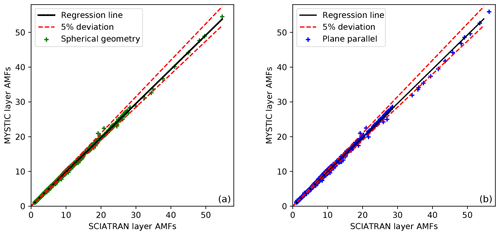Figure 2Scatter plots of MYSTIC 1D-layer AMFs computed with (a) spherical and (b) plane-parallel geometries against 1D-layer AMFs computed with SCIATRAN (a) spherical and (b) plane parallel for 67 MAX-DOAS scenarios with 17 layers (1139 points). The solid black lines are the respective regression fits to the points.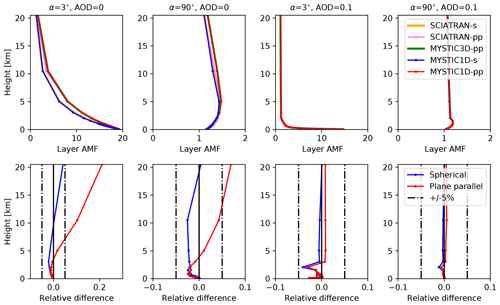Figure 3Upper row: MAX-DOAS AMF profiles for MYSTIC 1D spherical geometry (s), 1D plane-parallel geometry (pp) and 3D plane-parallel geometry (pp) for two selected elevation angles of 3 and 90, a SZA of 20, with and without aerosol for radiation at 577 nm. Corresponding profiles computed with the SCIATRAN RTM are shown for comparison. Lower row: profile of relative differences of MYSTIC and SCIATRAN results in spherical (s) and plane-parallel geometry (pp) .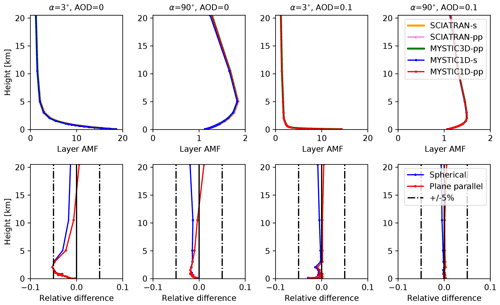Figure 4Upper row: MAX-DOAS AMF profiles for MYSTIC 1D spherical geometry (s), 1D plane-parallel geometry (pp) and 3D plane-parallel geometry (pp) for two selected elevation angles of 3 and 90, a SZA of 20, with and without aerosol for radiation at 360 nm. Corresponding profiles computed with the SCIATRAN RTM are shown for comparison. Lower row: profile of relative differences of MYSTIC and SCIATRAN results in spherical (s) and plane-parallel geometry (pp) .

To illustrate the differences in AMF profiles between the two RTMs, we selected four scenarios with a wavelength of 577 nm, because at this wavelength we observe comparatively large differences between the two models. To illustrate a usual scenario with low difference, we also selected the same scenarios but with a 360 nm wavelength. The upper row of Fig. 3 (scenario at 577 nm) and Fig. 4 (scenario at 360 nm) shows MYSTIC 1D-layer AMF profiles for the selected scenarios with a low elevation angle of 3 and a high elevation angle of 90 (zenith) without and with aerosols, respectively. For comparison, the corresponding profiles computed with SCIATRAN are also shown. The lower row presents the relative differences between MYSTIC and SCIATRAN. Since plane-parallel and spherical modes have different geometrical assumptions, we compare plane-parallel models and spherical models separately.

In the upper atmosphere, the 1D-layer AMFs decrease with altitude in all scenarios (Figs. 3 and 4), because the atmospheric density is decreasing, which lowers the amount of scattering and, correspondingly, the mean photon path length. In the lowest layers, however, the profile shapes are different for the two elevation angles with a rapid decrease with altitude in the low elevation angle scenarios and a local maximum between 2 and 5 km in the high elevation angle scenarios. This local maximum is caused by multiple scattering, which contributes to the horizontal light paths in those layers. The reduction towards the surface in the latter scenarios is due to the low surface albedo. For an elevation angle of 3, AMFs are high close to the ground because of the long light path in the layers due to the low elevation angle. Since aerosols increase scattering, photon path lengths and correspondingly 1D-layer AMFs are low in the lowest 2 km, when an aerosol layer is present.

1D-layer AMFs computed with spherical and plane-parallel geometry show noticeable differences for long wavelengths and low aerosol extinction, especially at altitudes above 5 km where extinction coefficients are small (see upper- and lower-left part in Fig. 3). In plane-parallel geometry, if one of these photons is traveling horizontally, it will strongly contribute to increase the mean photon path in that specific layer. In spherical mode, the same photon would change layer because of the curved atmospheric layers, and therefore its contribution to the mean photon path will be divided between the crossed layers. Furthermore, in a curved atmosphere, the zenith angle of the photon, which was initially traveling horizontally, will increase. At low altitude, these effects are smaller, and, conversely, 1D-layer AMFs computed with spherical and plane-parallel geometry agree better (mostly <5 %).

AMF profiles calculated with MYSTIC generally agree very well with those calculated with SCIATRAN with relative differences mostly smaller than 5 %. However, significant differences (up to 23 % relative difference) are seen between the plane-parallel solutions of the two models above 5 km for the scenarios without aerosols at 577 nm (Fig. 3). In contrast to the plane-parallel case, the spherical solution of MYSTIC is in good agreement with the spherical solution of SCIATRAN. The difference between SCIATRAN plane parallel and MYSTIC plane parallel is attributed to the different solution methods of the radiative transfer equation. A possible explanation is the following: in discrete ordinate methods, the directions of the radiation field are discretized and do not include the exact horizontal direction, for which in plane-parallel geometry the photon path length becomes extremely large in an optically thin medium like the higher atmosphere. In a Monte Carlo model, this horizontal direction is included; therefore the 1D-layer AMF might be larger. This hypothesis could be tested by including more streams (discrete directions) in SCIATRAN and verifying if the solution approaches the higher AMFs from the MYSTIC solution.

The simulations for the same scenarios but with 360 nm wavelength agree very well with SCIATRAN for both spherical and plane-parallel geometries (relative difference <5 %). The differences mentioned above are much smaller at this wavelength because atmospheric scattering events increase with lower wavelength and thus, prevent those very long photon paths. We also investigated a scenario with a wavelength of 440 nm, which is a typical wavelength of the window used for NO2 fitting (see Fig. S4 in the Supplement), for which MYSTIC and SCIATRAN also agree very well (<5 % relative difference), but as for simulations at 577 nm discussed above, the simulations at 440 nm show significant differences between plane-parallel and spherical geometry for layers above 5 km. These differences are, however, smaller than at 577 nm because the optical thickness of Rayleigh scattering is higher at 440 nm.

Overall, MYSTIC agrees very well with SCIATRAN with differences mainly smaller than 5 %. An exception is the high elevation scenario without aerosols, where the plane-parallel solutions of MYSTIC and SCIATRAN differ by up to 23 % for a wavelength of 577 nm at altitudes above 5 km. It should be noted that for these cases the 1D-layer AMFs are very small, and therefore the absolute differences, which are relevant for most applications, are also small. The 1D-layer AMFs computed with MYSTIC also agree very well with the other models presented in . Differences larger than 5 % are mainly attributable to differences between plane-parallel and spherical solutions (see the Supplement). When comparing MYSTIC with the mean of the models, 88.3 % of the compared points are within a relative difference of 5 % for spherical geometry, 81.5 % for plane-parallel geometry, and 97.5 % for the mean of plane-parallel and spherical geometry. The mean of spherical and plane-parallel geometry agrees best because the models in represents a mixture of spherical and plane-parallel solutions.

4 3D-box AMFs for MAX-DOAS observations

MAX-DOAS is a ground-based passive remote sensing technique allowing the retrieval of vertical concentration profiles of trace gases and aerosols . Information about the vertical distribution is obtained by measuring spectra at a prescribed sequence of elevation angles. Observations at different elevation angles have different sensitivity to the concentration in a given vertical layer. The 3D-box AMFs as computed by MYSTIC are particularly suitable to illustrate this, because 3D-box AMFs are a direct representation of the spatial distribution of the sensitivity of the measurements.

To illustrate the 3D distribution of 3D-box AMFs for a typical MAX-DOAS measurement, we simulated 3D-box AMFs at 450 nm for two scenarios with low and high aerosol optical depth, which correspond to a visibility of 50 and 10 km in the planetary boundary layer (PBL), respectively. A value of 450 nm is a typical wavelength for light absorption by NO2. The instrument points northwards with an azimuth angle of 180 and an elevation angle of 5. The solar azimuth angle (SAA) is 344.7 (164.7 relative azimuth angle), and the solar zenith angle (SZA) is 24.6. The MYSTIC input file is provided in the Supplement.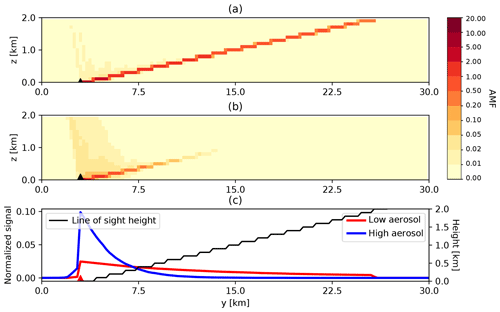Figure 5Cross section of 3D-box AMFs for a MAX-DOAS scenario with an instrument (black triangle) at the ground (z=0 km, x=20 km, y=3 km) pointing northwards and slightly upwards at a viewing angle of 5. The sun is at an azimuth angle of 344.7 and a zenith angle of 24.6. The relative azimuth angle between sun and viewing direction is 164.7. AMFs were simulated with two aerosol scenarios: a rural-type aerosol representative of spring–summer conditions in the aerosol layer (0–2 km), with a visibility of (a) 50 km and a visibility of (b) 10 km and a background aerosol above 2 km. Decay of vertically integrated AMFs with distance to the instrument is visualized (c) for the same scenarios with standard (red) and high aerosols (blue) as in panels (a) and (b). The altitude of the line of sight as a function of distance is shown in black.

Figure 5a and b show the 3D-box AMFs in the plane of the line of sight of the instrument for the two scenarios. In both cases, 3D-box AMFs are highest along the line of sight and reduce with distance from the instrument. Most of the photons collected by the instrument experienced a single scattering into the line of sight of the instrument. With increased aerosol amount (visibility of 10 km), photons scattered into the line of sight far away from the instrument have a high chance of being scattered out again. As a result, the sensitivity rapidly (within a few kilometers) decreases along the line of sight with increasing distance from the instrument. Multiple scattering becomes more important in this scenario, which explains the enhanced sensitivity to layers below and above the line of sight within a distance of up to 4 km of the instrument. The decrease in AMF with distance is further illustrated for the two scenarios in Fig. 5c, which shows the vertically integrated AMFs (in the aerosol layer) as a function of distance y to the instrument normalized with AMFs integrated horizontally in y direction. The figure also shows the height of the main optical path as a function of y.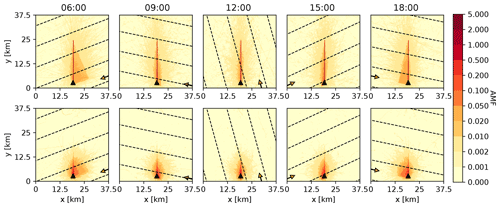Figure 6Top: vertically integrated 3D-box AMFs in the PBL (z<2.0 km) for an instrument at the ground pointing northwards with an instrument zenith angle of 5 for different times of the day on the 21st of June in Zurich. Solar zenith angles are 77.4, 47.5, 24.6, 38.6 and 68.5, and solar azimuth angles are 249.0, 281.5, 344.7, 65.2 and 101.7. The arrows point away from the sun, and the dashed lines show the direction of photons coming from the sun. AMFs were simulated with a rural-type aerosol representative of spring–summer conditions in the aerosol layer (0–2 km) with a visibility of 50 km and a background aerosol above 2 km. Bottom: same as above but for a scenario with increased aerosol (visibility of 10 km).

To illustrate the horizontal spread of the sensitivity of the MAX-DOAS measurements in the PBL, Fig. 6 shows horizontal distributions of vertically integrated 3D-box AMFs (0–2 km) for the same scenarios with low (top row) and high (bottom row) aerosols and for five different sun positions corresponding to different times of the day on 21 July in the city of Zurich. The horizontal distribution of AMFs shows high values not only along the line of sight of the instrument but also in a surrounding region, which is up to a few kilometers wide. This region is wider for larger relative azimuth angles and is inclined towards the direction of the sun. The simulations show not only that the MAX-DOAS measurements are sensitive to NO2 along the line of sight but also that they are also influenced by neighboring regions a few kilometers away.

For the different scenarios, we evaluated which part of the signal originated from a 0.25 km wide region centered on the northward pointing line of sight (referred to as main line in the following) and which part crossed boxes outside this range. For the low-aerosol scenario, between 63 % and 70 % originated from the main line. Thus, up to 37  % of the signal originated from photons crossing neighboring boxes. For the high-aerosol scenario with enhanced scattering, the part of the signal originating from the main line was correspondingly lower, between 30 % and 41 %. The lower values correspond to the scenarios with higher relative azimuth angles.

Depending on the viewing direction of the instrument relative to the position of nearby emission sources, this temporally varying spatial sensitivity could introduce a diurnal cycle in the measurement even when the trace gas concentration field was constant in time. Understanding the horizontal distribution of the sensitivity to NO2 and its variation in time is thus particularly important for the interpretation of MAX-DOAS observations in polluted regions like cities with strong NO2 gradients, for which 3D-box AMFs can be a valuable tool.

5 3D-box AMFs for airborne observations

In this section, we demonstrate the effect of the spatial variability in 3D-box AMFs on airborne NO2 imaging spectroscopy. For this purpose, we simulated a NO2 plume emitted from a stack to generate a scenario with a distinct three-dimensional trace gas structure. An airborne spectrometer was then assumed to fly parallel to the plume axis and to sample the plume in the across-track direction (see dashed lines in Fig. 8). We illustrate the distinct 3D-structure of the sensitivity of the measurements to NO2 (as represented by the 3D-box AMFs) and demonstrate the limitations of using 1D-layer AMFs for such observations.

## 5.1 Synthetic observations of a NO2 stack emission plume

The NO2 plume was computed with the Graz Lagrangian dispersion Model (GRAL) (Oettl2015) for a 262.5 m tall stack located at x=1.9 km and y=1.3 km. NO2 molecules were released at this altitude at a constant rate of 40 kg h−1. NOx chemistry was ignored for simplicity. The model domain had a size of 4 km × 4 km and extended from the surface to 21 km altitude. The simulated NO2 was sampled on an output grid with a 100 m horizontal resolution and 20 vertical levels with 25 m resolution from 0 to 500 m. For the simulation we assumed neutral atmospheric stability and southerly wind with a speed of 5 m s−1 at 12 m above ground. The full vertical wind profile is generated within the model based on similarity theory. The NO2 background from the US Standard Atmosphere was added to the simulated NO2 field, which was extended to 21 km altitude (see vertical resolution profile in the Supplement). The resulting NO2 VCDs are shown in Fig. 8a. In the following, the simulated NO2 concentration field and the corresponding NO2 VCDs are referred to as the true NO2 field and as the true total VCD, respectively. The true VCD will be used as a reference to demonstrate the limitations of 1D radiative transfer calculations.

Using MYSTIC, we computed the SCDs that would be observed from an airborne push-broom spectrometer flying parallel to the plume axis from south to north at an altitude of 6 km. The field of view in the across-track direction of the instrument covers the full x-direction of the model domain. The SCDs were obtained by computing 3D-box AMFs for each single observation (i.e., for each ground pixel) and multiplying these AMFs with the 3D NO2 field from the simulation (which corresponds to the numerator in Eq. 5).

As an example, Fig. 7 illustrates the 3D-box AMFs for an instrument pointing downwards at a zenith angle of 4.8 and an azimuth angle of 90. The sun is placed in the west (SAA=90) at a SZA of 20 – i.e., the instrument is facing the sun. The figure shows the 2D cross section of 3D-box AMFs in the principal plane of the observations, which aligns with the xz plane in this geometry. Figure 7b and c show the horizontally and vertically integrated 3D-box AMFs, i.e., layer and column AMFs, respectively. The layer AMFs are identical to 1D-layer AMFs. The 3D-box AMFs are high along the line of sight of the instrument and largest just below the aircraft. Most photons travel directly along the geometric path from the sun to the ground pixel and then to the instrument. Although 3D-box AMFs are highest along the geometric path due to the relatively bright surface, a non-negligible fraction of photons is scattered into the line of sight without reaching the surface, leading to an increase in 3D-box AMFs within a parallelogram bounded by the line of sight and the position of the sun.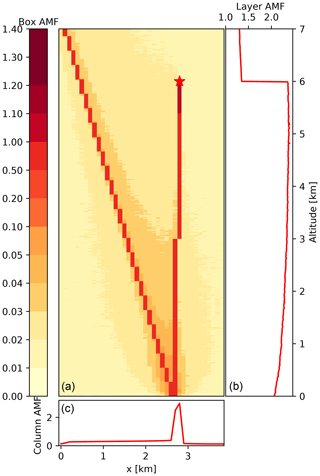Figure 7The 3D-box AMFs cross section at y=1.4 km for the aircraft scenario presented in this section. Aircraft (red star) placed at z=6 km, x=2.9 km and y=1.4 km pointing eastwards. The sun is at SAA=90 (west) with a SZA of 20. (b) Vertical profile of horizontally integrated AMFs (1D-layer AMFs). (c) Horizontal profile of vertically integrated AMFs (column AMFs). The default properties are a rural-type aerosol in the PBL, background aerosol above 2 km, spring–summer conditions and a visibility of 50 km.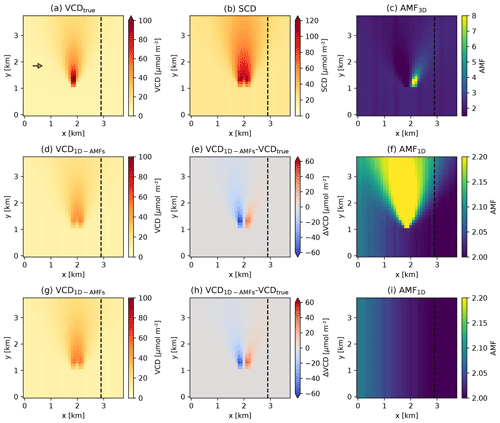Figure 8Airborne remote sensing of an NO2 plume emitted from a 262.5 m tall stack located at x=1.9 km and y=1.3 km. The aircraft flies at an altitude of 6 km from south to north at x=2.9 km (dashed line) parallel to the plume axis and samples the plume in the across-track direction. The sun is located in the west (small arrow in panel a) at a zenith angle of 40. The panels show (a) simulated (true) NO2 VCDs, (b) synthetic SCDs computed from the simulated NO2 distribution by applying 3D-box AMFs and (c) 3D-box AMFs computed with MYSTIC. The second row shows (d) VCDs calculated from the SCDs using 1D-layer AMFs and the “true” NO2 profile above the ground pixel pointed by the instrument, (e) the difference between calculated and true VCDs, and (f) total AMFs from the MYSTIC 1D module. The third row (g–i) shows the same as panels (d)(f) but using the background NO2 profile to compute AMFs.

The column AMFs (Fig. 7c) are highest close to the instrument and decrease with distance to the instrument in the x direction due to atmospheric scattering. After the “reflection point”, values continue to decrease with distance to the instrument but at a lower rate. Due to periodic boundaries this decrease continues on the right of the instrument (x≥3.9 km). Layer AMFs (i.e., 1D-layer AMFs) (Fig. 7b) are highest directly below the instrument. They change by a factor of 2 at the altitude of the aircraft because layers below are crossed (at least) twice by the photons, while layers above are only crossed once.

3D-box AMFs and corresponding SCDs were computed for four different solar zenith angles and four different relative azimuth angles between the sun and the plume axis (and flight direction). We used a default aerosol scenario with a rural-type aerosol representative of spring–summer conditions in the PBL (0–2 km) and a background aerosol above 2 km (visibility of 50 km in the PBL). The parameters used for the AMF calculation are summarized in Table 1. Note that with perfect knowledge of the relative NO2 distribution, the true total VCD could be reproduced exactly from the SCDs using 3D radiative transfer calculations. The computational cost of calculating 3D-box AMFs is considerably larger than for 1D-layer AMFs. The computational time for calculating 3D-box AMFs for the scenarios here (see Table 1 with SZA=20, SAA=90, VAA=90 and VZA=2) is around 218 s with 1 million photons using a single core of our local machine (Intel Xeon W-2175 CPU @ 2.5 GHz). The computational time for the corresponding 1D-layer AMFs is only about 4 s with 1 million photons. Note, however, that even less photons would be sufficient to obtain a similar noise level as for the 3D-box AMFs.

The SCDs computed for the scenario with the sun illuminating the scene from the west at a solar zenith angle of 40 are presented in Fig. 8b. The SCDs are larger than the VCDs (panel a) because the AMFs (panel c) are generally larger than 1. The SCD plume is wider and shifted towards the east compared to the VCD plume. The widening is due to both geometric effects and atmospheric scattering. Geometric effects are caused by the fact that photons following the main geometric path from the sun to the surface and to the instrument may traverse the plume either on the way from the sun to the surface or from the surface to the instrument (or both). These two pathways are separated horizontally. For high solar zenith angles (here SZA=40) this leads to two SCD maxima close to the source as seen in Fig. 8b. The westerly maximum corresponds to the direct observation of the plume (photons reflected by the surface pass the plume on the direct way to the aircraft), whereas the easterly maximum corresponds to its mirror image (photons first travel through the plume before they get reflected at the surface and reflected to the aircraft). This is further illustrated in Fig. 9, where two of the three illustrated direct paths (i.e., three viewing zenith angles) cross the NO2 maximum – main photon path (1) and (3) in Fig. 9. The main photon path for the observation angle (2) in Fig. 9 misses the plume maximum, which is why total SCD is lower for this observation. Atmospheric scattering leads to an additional horizontal smoothing of the plume, but in the case of a medium-high surface albedo of 0.2, the geometric effects dominate.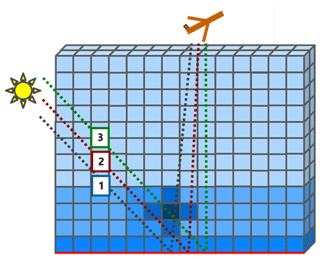Figure 9Schematic of the across-track measurement by the aircraft measuring a NO2 plume (dark blue corresponding to high NO2 concentrations) with three main photon paths for three measurement geometries (1, 2, 3).

## 5.2 Limitations of VCDs calculated from 1D-layer AMFs

For each scenario, total AMFs were also computed from 1D-layer AMFs, which requires a NO2 profile (Eq. 4). The most obvious approach is to use the true NO2 profile above the ground pixel the instrument is pointing towards, which is based on the idea that the AMF is used to convert an SCD to a VCD above a ground pixel (Fig. 8d, e, f). Alternatively, a NO2 background profile from the US Standard Atmosphere was used for each ground pixel, which assumes that no information on the spatial variability in NO2 is available (Fig. 8g, h, i).

Figure 8f and i show the total AMFs computed with the true and background NO2 profile, respectively. In both cases, AMFs increase with distance from the aircraft due to the increasing viewing zenith angle. For the true NO2 profiles, AMFs are higher inside the plume. This can be explained by the fact that the measurements are more sensitive to NO2 inside the plume than to the background NO2 outside because the plume is located at an altitude where the 1D-layer AMFs are higher.

Figure 8d and g show the VCDs obtained by dividing the true SCDs in Fig. 8b by the 1D-layer AMFs in Fig. 8f and i, respectively. Since geometric distortions and horizontal smoothing due to scattering cannot be corrected for when using a 1D radiative transfer model, all structures seen in the SCDs are essentially preserved in the VCDs, including the double peak structure, the widening of the plume and the horizontal displacement. Figure 8e and h show the differences in these VCDs from the true VCDs. In both cases, the location of the plume is shifted towards the aircraft relative to the true position. Within the maximum of the plume, this displacement leads to an underestimation of the true VCDs by −60.8µmol m−2 when using the NO2 profile (Fig. 8e) above the ground pixel and by −54.6µmol m−2 when using the constant NO2 profile (Fig. 8h).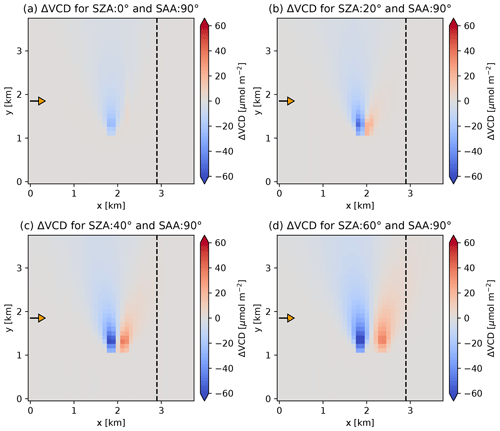Figure 10Absolute difference between total VCD from synthetic SCD and 1D box AMF with solar zenith angles (SZA) of (a) 0, (b) 20, (c) 40 and (d) 60 and the true total VCD.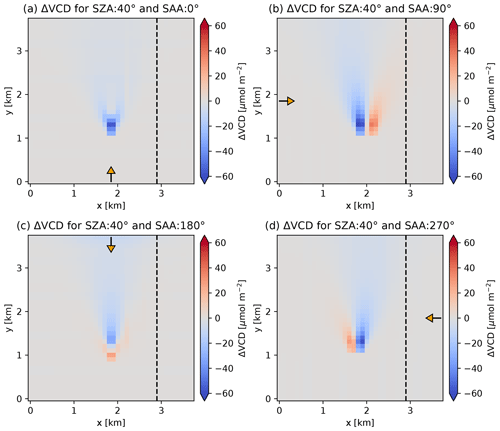Figure 11Absolute difference between total VCD from synthetic SCD and 1D box AMF with solar azimuth angle of (a) 0, (b) 90, (c) 180 and (d) 270 and the true total VCD.

The displacement of the calculated VCD plume and the magnitude of the bias depend on the position of the sun as demonstrated in Figs. 10 and 11. The shift increases with increasing SZA due to the geometric effects explained earlier. The relative azimuth angle between the viewing direction and the sun also plays a critical role. The displacement is smaller when the aircraft is flying directly away from the sun (SAA=0) or towards the sun (SAA=180) and the sun illuminates the scene along the plume axis, but even in these cases it is not negligible. Biases are typically larger when the spatial displacement is large.

## 5.3 Plume flux estimation

A possible application of airborne imaging spectroscopy is the estimation of NO2 emissions from point sources. Measurements from airborne spectrometers have been used, for example, to estimate CO2 emissions from power plants or CH4 emissions from coal mine ventilation shafts . The emissions can be estimated using a mass-balance approach by integrating the NO2 VCD enhancement above the background across the plume and multiplying this integral (referred to as line density in the following) with a mean wind speed to obtain a flux. The flux is equivalent to the source strength under the assumption of steady-state conditions.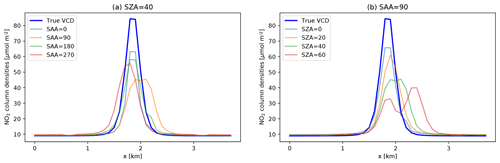Figure 12Plume VCD cross section at y=1.6 km (0.3 km downstream of the plume) for (a) the sun at SZA=40 with different SAAs and (b) for the sun in the west with different SZAs.

We computed line densities 300 m downstream of the source for the true VCD field and for fields computed with 1D-layer AMFs for different solar zenith and azimuth angles. The VCD cross sections are shown in Fig. 12. The line densities were multiplied with a wind speed of 9.1 m s−1, which is the wind speed at the stack height of 262.5 m in the GRAL simulation.

Table 2Estimated NO2 emissions from the retrieved VCD fields obtained from 1D-layer AMFs under different solar zenith angle (SZA) and solar azimuth angles (SAA).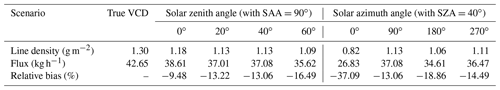Table 2 summarizes the computed line densities and fluxes for the different scenarios. In all scenarios, emissions were significantly underestimated by 9 %–37 % (relative to the true VCD) depending on the solar azimuth and zenith angle. Note that the emission estimation for the true VCD is slightly higher than the emission input for the dispersion model due to simplification of the mass-balance approach, which does not account for the vertical variability in wind speeds across the plume. The bias in the plume emission estimation using 1D-layer AMFs generally increases with solar zenith angle. This bias also depends on the solar azimuth angle. The largest bias occurs, when the SAA is 0 or 180, i.e., the instrument is flying towards and away from the sun.

6 Conclusions

This study demonstrates the importance of 3D radiative transfer effects for a range of trace gas remote sensing applications such as ground-based MAX-DOAS and airborne imaging spectroscopy. To study these effects, 1D-layer and 3D-box AMFs were implemented in the Monte Carlo solver MYSTIC of the libRadtran RTM. The computation of AMFs is a central component in most trace gas retrieval algorithms to convert observed SCDs into VCDs, but so far these algorithms were limited to 1D RTMs. In the case of a horizontally homogeneous atmosphere and in plane-parallel geometry, the 3D-box and 1D-layer AMFs perfectly agree within the statistical noise of the Monte Carlo method. They also agree very well with 1D-layer AMFs calculated with other RTMs presented in a previous model intercomparison study by .

The importance of 3D effects was demonstrated for two examples. For a ground-based MAX-DOAS instrument, we showed that 3D-box AMFs are highest along the line of sight of the instrument (representing photons that have mostly scattered only once) but that the contribution from outside is not negligible and depends on sun position and aerosol optical depth. The spatial distribution of the vertically integrated 3D-box AMFs depends on the sun position, which can be important for interpreting MAX-DOAS observations, especially in urban areas or, more generally, in the vicinity of pollution sources. The spatial variability in the NO2 distribution in the context of the MAX-DOAS instrument can affect the retrieval differently at different times of the day.

As second example, trace gas retrievals were studied for an airborne imaging spectrometer using simulations of a NO2 plume emitted by a stack. We showed that when using 1D-layer AMFs, the NO2 VCDs in the plume were significantly underestimated (up to 58 %) and that the position of the plume was artificially shifted towards the aircraft. Furthermore, integrals of the NO2 enhancement in the across-plume direction (line densities) were also biased, which results in an underestimation of the NO2 emissions from the stack when using a mass-balance approach. Using 1D-layer AMFs induces systematic errors even if the NO2 profile above the ground pixels is known accurately, because a 1D RTM fails to properly represent the complex light path, which is required if the trace gas field is not horizontally homogeneous.

Our study showed that even for simple examples, 3D effects are not negligible if the trace gas field has a high spatial variability. This finding is particularly relevant for ground-based and airborne remote sensing in cities, where considering 3D effects is likely indispensable to reduce systematic errors. This will be addressed in a followup study where the potential impact of 3D radiative transfer effects on the horizontal smoothing of the retrieved trace gas fields will also be studied. The 3D effects are also important for tomographic inversion where the application of 3D-box AMFs will minimize errors caused by the use of pure geometric assumptions. The high spatial resolution of the next generation of satellite instruments might make it necessary to also consider 3D effects for space-based trace gas remote sensing. Especially when considering imaging spectrometers with very high spatial resolution to estimate emissions (e.g., Strandgren et al.2020), 3D radiative transfer effects should be considered and studied. However, since 3D radiative transfer calculations are computationally expensive, efficient methods need to be developed for operational applications that provide an appropriate balance between accuracy and computational cost. To fully benefit from 3D-box AMFs, 3D radiative transfer calculations require high-resolution 3D distributions of trace gases and aerosols to calculate the total AMF. Such fields are generally difficult to obtain. In a followup study we plan to use 3D NO2 fields from a building-resolving urban air quality model with a detailed representation of both near-surface and elevated (stack) emission sources to further analyze the added value of 3D-box AMFs. On the other hand, measuring different azimuth angles with a MAX-DOAS instrument could be used to constrain the 3D fields of trace gases (e.g., Dimitropoulou et al.2019).

Code availability

The libRadtran package including the 1D version of MYSTIC is freely available on http://www.libradtran.org ; the 3D MYSTIC code is available upon request to Claudia Emde (claudia.emde@lmu.de), and the other used codes are available upon request to the corresponding author.

Data availability

The data used for this study are available at https://doi.org/10.5281/zenodo.3948112 .

Supplement

Author contributions

MS implemented the 3D-box AMF module and validated the implementation, designed and simulated the 3D scenarios, and wrote the paper with input from all coauthors. CE implemented 1D-layer AMF module, implemented the 3D-box AMFs together with MS, provided assistance with study design and reviewed the paper. TW provided the 1D-layer AMF data used for the validation and reviewed the paper. DB, BB and AB provided critical feedback to the study and reviewed the paper; RM conducted and provided the GRAL simulation; GK supervised the study, designed together with MS the case studies and reviewed the paper.

Competing interests

The authors declare that they have no conflict of interest.

Financial support

This research has been supported by the Swiss National Science Foundation (SNSF, grant no. 172533).

Review statement

This paper was edited by Folkert Boersma and reviewed by Frederik Tack and one anonymous referee.

References

Berchet, A., Zink, K., Muller, C., Oettl, D., Brunner, J., Emmenegger, L., and Brunner, D.: A cost-effective method for simulating city-wide air flow and pollutant dispersion at building resolving scale, Atmos. Environ., 158, 181–196, 2017. a

Berk, A., Anderson, G. P., Bernstein, L. S., Acharya, P. K., Dothe, H., Matthew, M. W., Adler-Golden, S. M., Chetwynd Jr., J. H., Richtsmeier, S. C., Pukall, B., Allred, C. L., Jeong, L. S., and Hoke, M. L.: MODTRAN4 radiative transfer modeling for atmospheric correction, in: Optical spectroscopic techniques and instrumentation for atmospheric and space research III, vol. 3756, 348–353, International Society for Optics and Photonics, Denver, CO, USA, 1999. a

Boersma, K. F., Eskes, H. J., Dirksen, R. J., van der A, R. J., Veefkind, J. P., Stammes, P., Huijnen, V., Kleipool, Q. L., Sneep, M., Claas, J., Leitão, J., Richter, A., Zhou, Y., and Brunner, D.: An improved tropospheric NO2 column retrieval algorithm for the Ozone Monitoring Instrument, Atmos. Meas. Tech., 4, 1905–1928, https://doi.org/10.5194/amt-4-1905-2011, 2011. a

Burrows, J. P., Weber, M., Buchwitz, M., Rozanov, V., Ladstätter-Weißenmayer, A., Richter, A., DeBeek, R., Hoogen, R., Bramstedt, K., Eichmann, K. U., Eisinger, M., and Perner, D.: The global ozone monitoring experiment (GOME): Mission concept and first scientific results, J. Atmos. Sci., 56, 151–175, 1999. a

Casaballe, N., Di Martino, M., Osorio, M., Ferrari, J., Wagner, T., and Frins, E.: Improved algorithm with adaptive regularization for tomographic reconstruction of gas distributions using DOAS measurements, Appl. Optics, 59, D179–D188, 2020. a

Deutschmann, T., Beirle, S., Frieß, U., Grzegorski, M., Kern, C., Kritten, L., Platt, U., Prados-Román, C., Puķı¯te, J., Wagner, T., Werner, B., and Pfeilsticker, K.: The Monte Carlo atmospheric radiative transfer model McArtim: Introduction and validation of Jacobians and 3D features, J. Quant. Spectrosc. Ra., 112, 1119–1137, https://doi.org/10.1016/j.jqsrt.2010.12.009, 2011. a, b

Dimitropoulou, E., Van Roozendael, M., Hendrick, F., Merlaud, A., Tack, F., Fayt, C., Hermans, C., and Pinardi, G.: One year of 3-D MAX-DOAS tropospheric NO2 measurements over Brussels, in: EGU General Assembly Conference Abstracts, EGU General Assembly Conference, 7–12 April 2019, Vienna, Austria, 5874, 2019. a

Emde, C. and Mayer, B.: Simulation of solar radiation during a total eclipse: a challenge for radiative transfer, Atmos. Chem. Phys., 7, 2259–2270, https://doi.org/10.5194/acp-7-2259-2007, 2007. a, b

Emde, C., Buras-Schnell, R., Kylling, A., Mayer, B., Gasteiger, J., Hamann, U., Kylling, J., Richter, B., Pause, C., Dowling, T., and Bugliaro, L.: The libRadtran software package for radiative transfer calculations (version 2.0.1), Geosci. Model Dev., 9, 1647–1672, https://doi.org/10.5194/gmd-9-1647-2016, 2016. a, b

Emde, C., Buras-Schnell, R., Sterzik, M., and Bagnulo, S.: Influence of aerosols, clouds, and sunglint on polarization spectra of Earthshine, Astron. Astrophys., 605, A2, https://doi.org/10.1051/0004-6361/201629948, 2017. a

Frankenberg, C., Platt, U., and Wagner, T.: Iterative maximum a posteriori (IMAP)-DOAS for retrieval of strongly absorbing trace gases: Model studies for CH4 and CO2 retrieval from near infrared spectra of SCIAMACHY onboard ENVISAT, Atmos. Chem. Phys., 5, 9–22, https://doi.org/10.5194/acp-5-9-2005, 2005. a

Frieß, U., Monks, P., Remedios, J., Rozanov, A., Sinreich, R., Wagner, T., and Platt, U.: MAX-DOAS O4 measurements: A new technique to derive information on atmospheric aerosols: 2. Modeling studies, J. Geophys. Res.-Atmos., 111, D14203, https://doi.org/10.1029/2005JD006618, 2006. a

Frins, E., Bobrowski, N., Platt, U., and Wagner, T.: Tomographic multiaxis-differential optical absorption spectroscopy observations of Sun-illuminated targets: a technique providing well-defined absorption paths in the boundary layer, Appl. Optics, 45, 6227–6240, 2006. a

Hendrick, F., Müller, J.-F., Clémer, K., Wang, P., De Mazière, M., Fayt, C., Gielen, C., Hermans, C., Ma, J. Z., Pinardi, G., Stavrakou, T., Vlemmix, T., and Van Roozendael, M.: Four years of ground-based MAX-DOAS observations of HONO and NO2 in the Beijing area, Atmos. Chem. Phys., 14, 765–781, https://doi.org/10.5194/acp-14-765-2014, 2014. a

Hönninger, G. and Platt, U.: Observations of BrO and its vertical distribution during surface ozone depletion at Alert, Atmos. Environ., 36, 2481–2489, 2002. a

Irie, H., Takashima, H., Kanaya, Y., Boersma, K. F., Gast, L., Wittrock, F., Brunner, D., Zhou, Y., and Van Roozendael, M.: Eight-component retrievals from ground-based MAX-DOAS observations, Atmos. Meas. Tech., 4, 1027–1044, https://doi.org/10.5194/amt-4-1027-2011, 2011. a, b

Iwabuchi, H.: Efficient Monte Carlo methods for radiative transfer modeling, J. Atmos. Sci., 63, 2324–2339, 2006. a

Iwabuchi, H. and Okamura, R.: Multispectral Monte Carlo radiative transfer simulation by the maximum cross-section method, J. Quant. Spectrosc. Ra., 193, 40–46, 2017. a

Kazahaya, R., Mori, T., Kazahaya, K., and Hirabayashi, J.-I.: Computed tomography reconstruction of SO2 concentration distribution in the volcanic plume of Miyakejima, Japan, by airborne traverse technique using three UV spectrometers, Geophys. Res. Lett., 35, L13816, https://doi.org/10.1029/2008GL034177, 2008. a

Krings, T., Gerilowski, K., Buchwitz, M., Reuter, M., Tretner, A., Erzinger, J., Heinze, D., Pflüger, U., Burrows, J. P., and Bovensmann, H.: MAMAP – a new spectrometer system for column-averaged methane and carbon dioxide observations from aircraft: retrieval algorithm and first inversions for point source emission rates, Atmos. Meas. Tech., 4, 1735–1758, https://doi.org/10.5194/amt-4-1735-2011, 2011. a

Krings, T., Gerilowski, K., Buchwitz, M., Hartmann, J., Sachs, T., Erzinger, J., Burrows, J. P., and Bovensmann, H.: Quantification of methane emission rates from coal mine ventilation shafts using airborne remote sensing data, Atmos. Meas. Tech., 6, 151–166, https://doi.org/10.5194/amt-6-151-2013, 2013. a

Krueger, A. J., Walter, L. S., Bhartia, P. K., Schnetzler, C. C., Krotkov, N. A., Sprod, I., and Bluth, G. J. S.: Volcanic sulfur dioxide measurements from the total ozone mapping spectrometer instruments, J. Geophys. Res.-Atmos., 100, 14057–14076, 1995. a

Lerot, C., Van Roozendael, M., Lambert, J.-C., Granville, J., Van Gent, J., Loyola, D., and Spurr, R.: The GODFIT algorithm: a direct fitting approach to improve the accuracy of total ozone measurements from GOME, Int. J. Remote Sens., 31, 543–550, 2010. a

Marchuk, G. I., Mikhailov, G. A., and Nazaraliev, M. A.: The Monte Carlo methods in atmospheric optics, Springer Series in Optical Sciences, Springer, Berlin, Germany, 1980. a

Marshak, A. and Davis, A.: 3D Radiative Transfer in Cloudy Atmospheres, Springer, Heidelberg, Germany, https://doi.org/10.1007/3-540-28519-9, 2005. a

Martin, R. V., Jacob, D. J., Chance, K., Kurosu, T. P., Palmer, P. I., and Evans, M. J.: Global inventory of nitrogen oxide emissions constrained by space-based observations of NO2 columns, J. Geophys. Res., 108, 4537, https://doi.org/10.1029/2003JD003453, 2003. a

Mayer, B.: Radiative transfer in the cloudy atmosphere, in: EPJ Web of Conferences, EDP Sciences, Les Ulis, France, vol. 1, 75–99, https://doi.org/10.1140/epjconf/e2009-00912-1, 2009. a

Mayer, B. and Kylling, A.: Technical note: The libRadtran software package for radiative transfer calculations – description and examples of use, Atmos. Chem. Phys., 5, 1855–1877, https://doi.org/10.5194/acp-5-1855-2005, 2005. a, b, c

Mayer, B., Emde, C., Gasteiger, J., and Kylling, A.: libRadtran, available at: http://www.libradtran.org, last access: 12 August 2020. a

McPeters, R. D., Frith, S., and Labow, G. J.: OMI total column ozone: extending the long-term data record, Atmos. Meas. Tech., 8, 4845–4850, https://doi.org/10.5194/amt-8-4845-2015, 2015. a

Mijling, B., van der A, R. J., and Zhang, Q.: Regional nitrogen oxides emission trends in East Asia observed from space, Atmos. Chem. Phys., 13, 12003–12012, https://doi.org/10.5194/acp-13-12003-2013, 2013. a

Nowlan, C. R., Liu, X., Leitch, J. W., Chance, K., González Abad, G., Liu, C., Zoogman, P., Cole, J., Delker, T., Good, W., Murcray, F., Ruppert, L., Soo, D., Follette-Cook, M. B., Janz, S. J., Kowalewski, M. G., Loughner, C. P., Pickering, K. E., Herman, J. R., Beaver, M. R., Long, R. W., Szykman, J. J., Judd, L. M., Kelley, P., Luke, W. T., Ren, X., and Al-Saadi, J. A.: Nitrogen dioxide observations from the Geostationary Trace gas and Aerosol Sensor Optimization (GeoTASO) airborne instrument: Retrieval algorithm and measurements during DISCOVER-AQ Texas 2013, Atmos. Meas. Tech., 9, 2647–2668, https://doi.org/10.5194/amt-9-2647-2016, 2016. a

Oettl, D.: Quality assurance of the prognostic, microscale wind-field model GRAL 14.8 using wind-tunnel data provided by the German VDI guideline 3783-9, J. Wind Eng. Ind. Aerod., 142, 104–110, https://doi.org/10.1016/j.jweia.2015.03.014, 2015. a

Palmer, P. I., Jacob, D. J., Chance, K., Martin, R. V., Spurr, R. J., Kurosu, T. P., Bey, I., Yantosca, R., Fiore, A., and Li, Q.: Air mass factor formulation for spectroscopic measurements from satellites: Application to formaldehyde retrievals from the Global Ozone Monitoring Experiment, J. Geophys. Res.-Atmos., 106, 14539–14550, 2001. a, b

Platt, U. and Stutz, J.: Differential optical absorption spectroscopy: principles and applications, Springer Verlag, Berlin, Heidelberg, Germany, 2008. a

Popp, C., Brunner, D., Damm, A., Van Roozendael, M., Fayt, C., and Buchmann, B.: High-resolution NO2 remote sensing from the Airborne Prism EXperiment (APEX) imaging spectrometer, Atmos. Meas. Tech., 5, 2211–2225, https://doi.org/10.5194/amt-5-2211-2012, 2012. a

Postylyakov, O.: Radiative transfer model MCC++ with evaluation of weighting functions in spherical atmosphere for use in retrieval algorithms, Adv. Space Res., 34, 721–726, 2004. a

Puķīte, J., Kühl, S., Deutschmann, T., Dörner, S., Jöckel, P., Platt, U., and Wagner, T.: The effect of horizontal gradients and spatial measurement resolution on the retrieval of global vertical NO2 distributions from SCIAMACHY measurements in limb only mode, Atmos. Meas. Tech., 3, 1155–1174, https://doi.org/10.5194/amt-3-1155-2010, 2010. a

Richter, A., Eyring, V., Burrows, J. P., Bovensmann, H., Lauer, A., Sierk, B., and Crutzen, P. J.: Satellite measurements of NO2 from international shipping emissions, Geophys. Res. Lett., 31, L23110, https://doi.org/10.1029/2004GL020822, 2004. a

Richter, A., Godin, S., Gomez, L., Hendrick, F., Hocke, K., Langerock, B., van Roozendael, M., and Wagner, T.: Spatial Representativeness of NORS observations, Tech. rep., Network of Remote Sensing Ground-Based Observations for the GMES Atmospheric Service, Report from Institute of Environmental Physics, University of Bremen, Bremen, Germany, available at: http://nors.aeronomie.be/projectdir/PDF/D4.4_NORS_SR.pdf (last access: 12 August 2020), 2013. a

Rozanov, A., Rozanov, V., Buchwitz, M., Kokhanovsky, A., and Burrows, J.: SCIATRAN 2.0 – A new radiative transfer model for geophysical applications in the 175–2400 nm spectral region, Adv. Space Res., 36, 1015–1019, 2005. a, b

Rozanov, V. V. and Rozanov, A. V.: Differential optical absorption spectroscopy (DOAS) and air mass factor concept for a multiply scattering vertically inhomogeneous medium: theoretical consideration, Atmos. Meas. Tech., 3, 751–780, https://doi.org/10.5194/amt-3-751-2010, 2010. a, b

Russell, A. R., Valin, L. C., and Cohen, R. C.: Trends in OMI NO2 observations over the United States: effects of emission control technology and the economic recession, Atmos. Chem. Phys., 12, 12197–12209, https://doi.org/10.5194/acp-12-12197-2012, 2012. a

Schaub, D., Brunner, D., Boersma, K. F., Keller, J., Folini, D., Buchmann, B., Berresheim, H., and Staehelin, J.: SCIAMACHY tropospheric NO2 over Switzerland: estimates of NOx lifetimes and impact of the complex Alpine topography on the retrieval, Atmos. Chem. Phys., 7, 5971–5987, https://doi.org/10.5194/acp-7-5971-2007, 2007. a

Schönhardt, A., Altube, P., Gerilowski, K., Krautwurst, S., Hartmann, J., Meier, A. C., Richter, A., and Burrows, J. P.: A wide field-of-view imaging DOAS instrument for two-dimensional trace gas mapping from aircraft, Atmos. Meas. Tech., 8, 5113–5131, https://doi.org/10.5194/amt-8-5113-2015, 2015. a

Schwaerzel, M., Emde, C., Brunner, D., Morales, R., Wagner, T., Berne, A., Buchmann, B., and Kuhlmann, G.: Dataset – Three-dimensional radiative transfer effects on airborne and ground-based trace gas remote sensing, Zenodo, https://doi.org/10.5281/zenodo.3948112, 2020. a

Solomon, S., Schmeltekopf, A. L., and Sanders, R. W.: On the interpretation of zenith sky absorption measurements, J. Geophys. Res., 92, 8311–8319, 1987. a

Spurr, R., Kurosu, T., and Chance, K.: A linearized discrete ordinate radiative transfer model for atmospheric remote-sensing retrieval, J. Quant. Spectrosc. Ra., 68, 689–735, 2001. a

Strandgren, J., Krutz, D., Wilzewski, J., Paproth, C., Sebastian, I., Gurney, K. R., Liang, J., Roiger, A., and Butz, A.: Towards spaceborne monitoring of localized CO2 emissions: an instrument concept and first performance assessment, Atmos. Meas. Tech., 13, 2887–2904, https://doi.org/10.5194/amt-13-2887-2020, 2020.  a

Tack, F., Merlaud, A., Iordache, M.-D., Danckaert, T., Yu, H., Fayt, C., Meuleman, K., Deutsch, F., Fierens, F., and Van Roozendael, M.: High-resolution mapping of the NO2 spatial distribution over Belgian urban areas based on airborne APEX remote sensing, Atmos. Meas. Tech., 10, 1665–1688, https://doi.org/10.5194/amt-10-1665-2017, 2017. a

United States Committee on Extension to the Standard Atmosphere: US Standard Atmosphere, vol. 76, National Oceanic and Atmospheric Administration, National Oceanic and Atmospheric Administration and United States, United States Air Force, Washignton, D.C., USA, 1976. a, b, c

Wagner, T., Dix, B. v., Friedeburg, C. v., Frieß, U., Sanghavi, S., Sinreich, R., and Platt, U.: MAX-DOAS O4 measurements: A new technique to derive information on atmospheric aerosols – Principles and information content, J. Geophys. Res.-Atmos., 109, D22205, https://doi.org/10.1029/2004JD004904, 2004. a

Wagner, T., Burrows, J. P., Deutschmann, T., Dix, B., von Friedeburg, C., Frieß, U., Hendrick, F., Heue, K.-P., Irie, H., Iwabuchi, H., Kanaya, Y., Keller, J., McLinden, C. A., Oetjen, H., Palazzi, E., Petritoli, A., Platt, U., Postylyakov, O., Pukite, J., Richter, A., van Roozendael, M., Rozanov, A., Rozanov, V., Sinreich, R., Sanghavi, S., and Wittrock, F.: Comparison of box-air-mass-factors and radiances for Multiple-Axis Differential Optical Absorption Spectroscopy (MAX-DOAS) geometries calculated from different UV/visible radiative transfer models, Atmos. Chem. Phys., 7, 1809–1833, https://doi.org/10.5194/acp-7-1809-2007, 2007. a, b, c, d, e, f, g, h, i, j, k, l, m, n, o, p

Wenig, M. O., Cede, A. M., Bucsela, E. J., Celarier, E. A., Boersma, K. F., Veefkind, J. P., Brinksma, E. J., Gleason, J. F., and Herman, J. R.: Validation of OMI tropospheric NO2 column densities using direct-Sun mode Brewer measurements at NASA Goddard Space Flight Center, J. Geophys. Res., 113, D16S45, https://doi.org/10.1029/2007JD008988, 2008. a

Wu, F. C., Xie, P. H., Li, A., Chan, K. L., Hartl, A., Wang, Y., Si, F. Q., Zeng, Y., Qin, M., Xu, J., Liu, J. G., Liu, W. Q., and Wenig, M.: Observations of SO2 and NO2 by mobile DOAS in the Guangzhou eastern area during the Asian Games 2010, Atmos. Meas. Tech., 6, 2277–2292, https://doi.org/10.5194/amt-6-2277-2013, 2013. a

Zhou, Y., Brunner, D., Hueglin, C., Henne, S., and Staehelin, J.: Changes in OMI tropospheric NO2 columns over Europe from 2004 to 2009 and the influence of meteorological variability, Atmos. Environ., 46, 482–495, https://doi.org/10.1016/j.atmosenv.2011.09.024, 2012. a